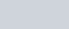• 14
•
•
•
•
•
•
•
14
Shares

# DERIVATION OF ELECTRIC POTENTIAL DUE TO A ISOLATED POINT CHARGE

In the previous article we have talked about electric potential and electric potential difference in detail. In this article we are going to derive an expression for electric potential due to an isolated point charge. So keep reading till end.

### DERIVATION OF THE EXPRESSION

Let P be the point at a distance r from the origin O at which we are interested to find the magnitude of electric potential due to a point charge +q.What will the electric potential due to charge +q at point P?. By the definition of electric potential, we know that electric potential at point P is measure of amount of work done in bringing a unit positive charge from infinity to point P, such that it doesn’t go in acceleration. Means it did not emmits any electromagnetic radiation.

As we know that work done is independent of the path choosen. So we choose a convenient path along the radial direction from the infinity to the point P without any acceleration.

Let A is the intermediate point on this path, where OA = x.
Then, the electrostatic force experienced by the unit positive charge at point A is-

(1)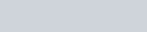The small work done in moving the unit charge through small distance dx from the point A to B is-

(2)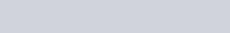Now the total work done in bringing unit positive charge from infinity to the point P is-

(3)From the definition of electric potential, this work done is equal to the electric potential at point P. Because if we divide this work done by the unit positive charge, then we got the same value as below.

(4)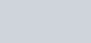So this is the required value of electric potential due to a point charge +q at the distance r.Electric potential due to a single positive charge, magenta colour show the electric potential. Central contours are equipotential lines. Image source : Wikipedia.org

A positively charged particles produces a positive Electric potential and a negatively charged particles produces a negative electric potential. In the derivation of electric potential, here we assume that electrostatic potential is zero at infinity.

The symmetry of the electrostatic potential due to a isolated single point charge is spherical in shape. If we observe the expression that we obtained then we will see that the electric potential is directly proportional to the electric charge (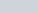) and it is inversely proportional to the distance r,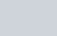, whereas the variation of electric field with distance r is the inversely proportional to the square of the distance r,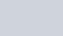The variation of electric potential and electric field is shown in the following graph.Variation of electric potential and electric field. Image source : sarthak eConnect

### FREQUENTLY ASKED QUESTIONS ON DERIVATION OF ELECTRIC POTENTIAL

1). Can electric potential be zero?

Ans- Yes, electric potential can be zero, if the point, where we wanted to find electric potential, is placed at infinity then then electric potential for infinity is zero. Put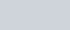in the expression of electric potential, we get the value zero. If you start bringing the charge from infinity towards the source charge then electric potential starts increasing from 0 to some value. Smaller the r greater the magnitude.

2). Can electric potential at a point be zero while electric field is not zero?

Ans- yes, it can be zero, if your point is placed on the equatorial line of electric dipole.

3). Under which condition electric field intensity at a point is zero but electric potential is not zero?

Ans- In the midpoint of electric dipole, the electric field intensity is zero but electric potential is non zero.

4). How do you find the electric potential of a point charge?

Ans- Any can find the electric potential of a point charge at any distance r by the below formula.